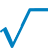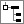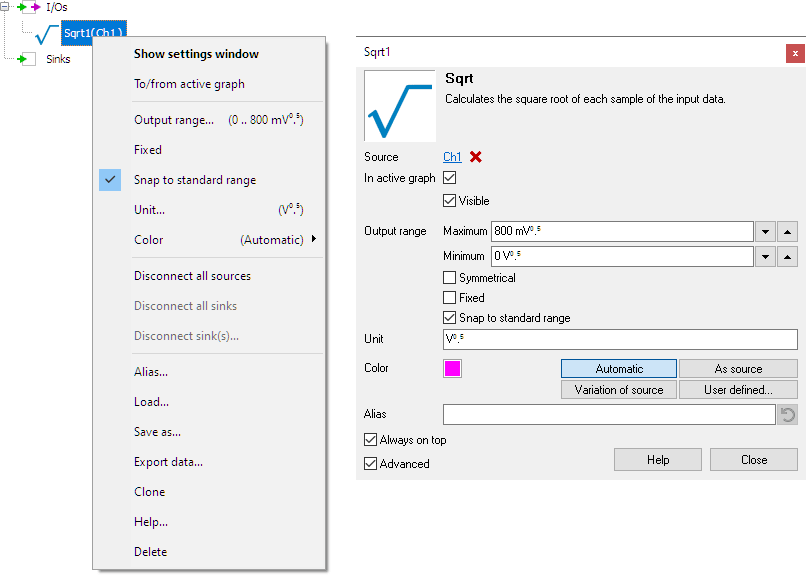# SQRTThe SQRT I/O calculates the square root of each sample of the source's data.

The typical application of the SQRT I/O is to use it as part of larger, more complex mathematical operations.

Hint: The SQRT-I/O can be used to calculate RMS values. Perform the following steps:
• Create a Multiply/Divide
• Create a Resampler, set its Method to Average and its Out/In ratio to output 1 sample
• Create a SQRT
• Drag your source onto the Multiply/Divide twice to calculate squared values of the source's data.
• Drag the Multiply/Divide onto the Resampler to calculate a running mean of the squares.
• Drag the Resampler onto the SQRT to get the RMS values.

## Common properties and actions

To control the behavior of the SQRT I/O, several properties and actions are available. These can be accessed through a popup menu which is shown when the I/O is right clicked in the Object Tree. The properties can also be accessed through its settings window which is shown when the I/O is double clicked in the Object Tree. To open the Object tree, click theShow object tree button.By default, the settings window only shows the most used settings. When Advanced is ticked, the extended window with all settings is shown. See also the program settings.

### Related information

#### Gain / Offset

The Gain / Offset I/O multiplies a signal with a constant gain factor and adds a constant offset.

The Add / Subtract I/O adds or subtracts data of two or more sources.

#### Multiply / Divide

The Multiply / Divide I/O multiplies or divides data of two ore more sources.

#### ABS

The ABS I/O takes the absolute value of each sample of the source's data.

#### Differentiate

The Differentiate I/O differentiates the source's data.

#### Integrate

The Integrate I/O integrates its source's data.

#### Logarithm

The Logarithm I/O calculates the logarithm of the source's data.

#### Resampler

The Resampler I/O resamples the source's data to decrease or increase the sample frequency (and record length with the same ratio) of a signal.